Welcome to LaserPlot

# LaserPlot Purpose

This Shiny application allows the user to explore a data file outputted from the Levitation Laser Furnace. Once loaded, the user can view the temperature vs time plot and download it as a pdf or png file. They also have the option to include linear regressions over the cooling or heating periods and their accompanying equations on the plot, where the slope represents the rate of change. The rates are also shown on the right, calculated by averaging the derivatives throughout the cooling or heating periods. The user also has the option to download a single-row table in csv format.

The file contains (1) sample name, (2) date, (3) gas A, (4) gas A percent, (5) gas B, (6) gas B percent, (7) gas flow, (8) heating duration, (9) holding duration, (10) mean hold temperature, (11) peak temperature reached, (12) cooling rate (regression), (13) cooling rate (averaged derivative), (14) heating rate (regression), (15) heating rate (averaged derivative), and (16) additional notes.

1. The sample name is determined by the file name without the file type suffix (.xls).

2. The date when the sample was run and the file was created.

3. The gas running through valve A.

4. The ratio of the gas running through valve A over the total gas flow (as a percentage).

5. The gas running through valve B.

6. The ratio of the gas running through valve B over the total gas flow (as a percentage).

7. The gas flow is pulled from the input file, but if the rate changed during levitation, this value can be adjusted by user input.

8. The heating duration marks the amount of time (in seconds) from which the heating rates are calculated.

9. The holding duration marks the amount of time (in seconds) beginning at the selected transition from heating to holding and ending on the last non-zero laser power entry.

10. The mean hold temperature is the mean temperature calculated during the holding duration.

11. The peak temperature is a calculated maximum.

12. The cooling rate (regression) is the rate determined by the linear regression from the moment the laser is shut off to the moment the temperature drops below 600 degrees Celsius.

13. The cooling rate (averaged derivative) is the rate calculated by the average of first derivatives from the moment the laser is shut off to the moment the temperature drops below 600 degrees Celsius.

14. The heating rate (regression) is the rate determined by the linear regression from the moment the temperature of the sample rises above 600 degrees Celsius and continues until a user-defined top time. This time will default to 2000 seconds. By defaulting to 2000, the maximum time for the heat rate will be equal to the moment that the laser shuts off.

15. The heating rate (averaged derivative) is determined by the average of first derivatives from the moment the temperature of the sample rises above 600 degrees Celsius and continues until a user-defined top time. This time will default to 2000 seconds. By defaulting to 2000, the maximum time for the heat rate will be equal to the moment that the laser shuts off.

16. The additional notes are optional text for the user to add (on the left side of the window) when viewing the plot.

# LaserPlot Use

LaserPlot is designed for the .xls file output from the Levitation Laser Furnace. These files have system information contained in the first 121 lines, which this Shiny application ignores.

IMPORTANT:

Each file must represent a single heating and cooling run. If the laser is turned on and off multiple times in a single file, the program will not function properly.

Also, based on how the program interprets the data file, the last entry in the file must have a laser power of zero.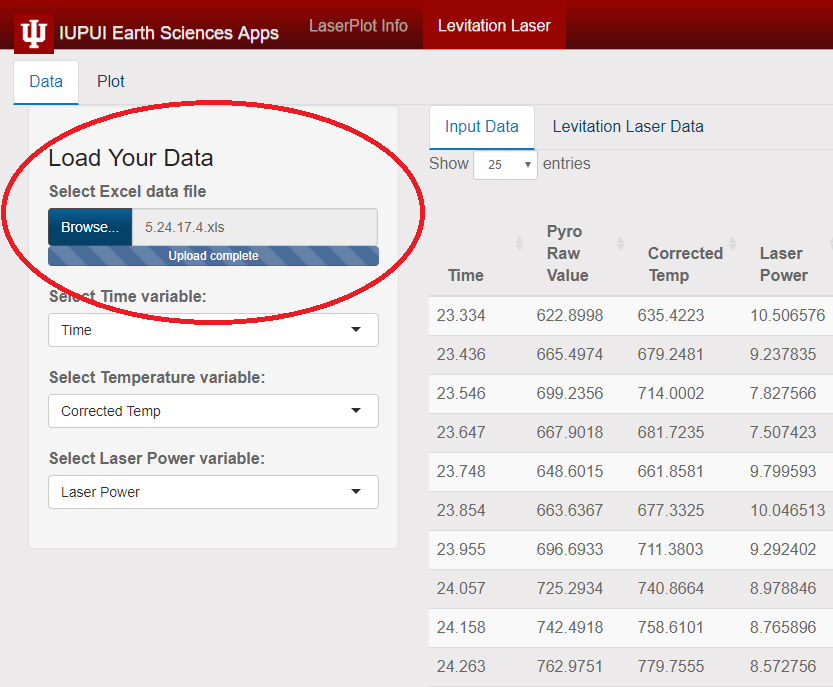Load data into LaserPlot by clicking the “Browse” button and selecting the file from its location on your computer.

### Selecting Variables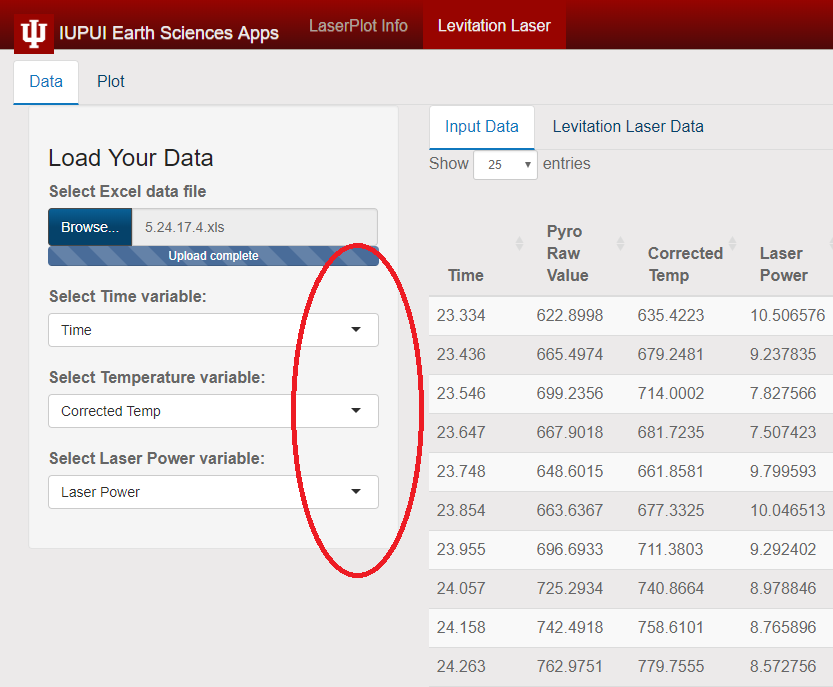The application automatically selects the Time, Corrected Temp, and Laser Power variables. These can be changed from the drop-down menu if desired.

### Input Data Tab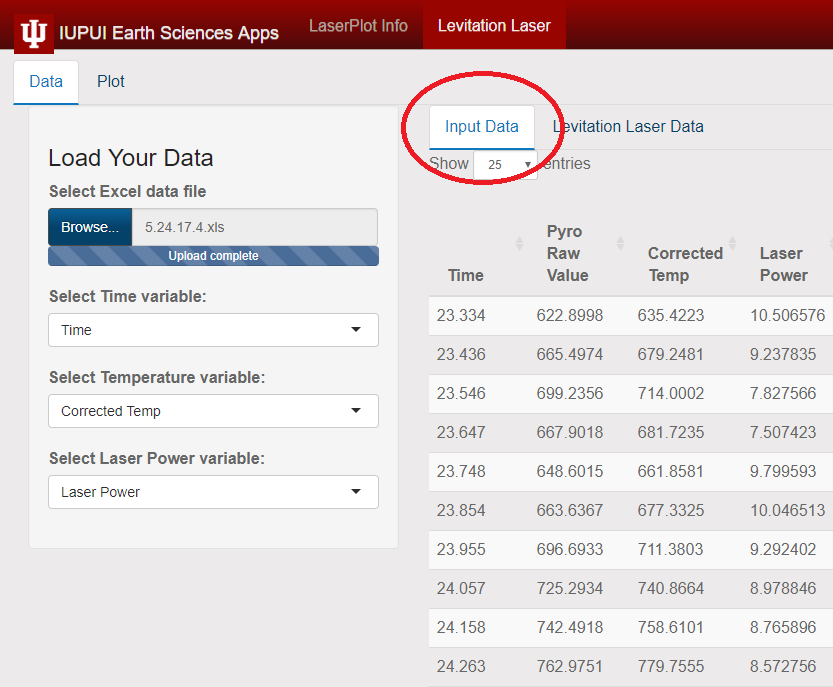The Input Data tab shows the raw data from the loaded data file.

### Levitation Laser Data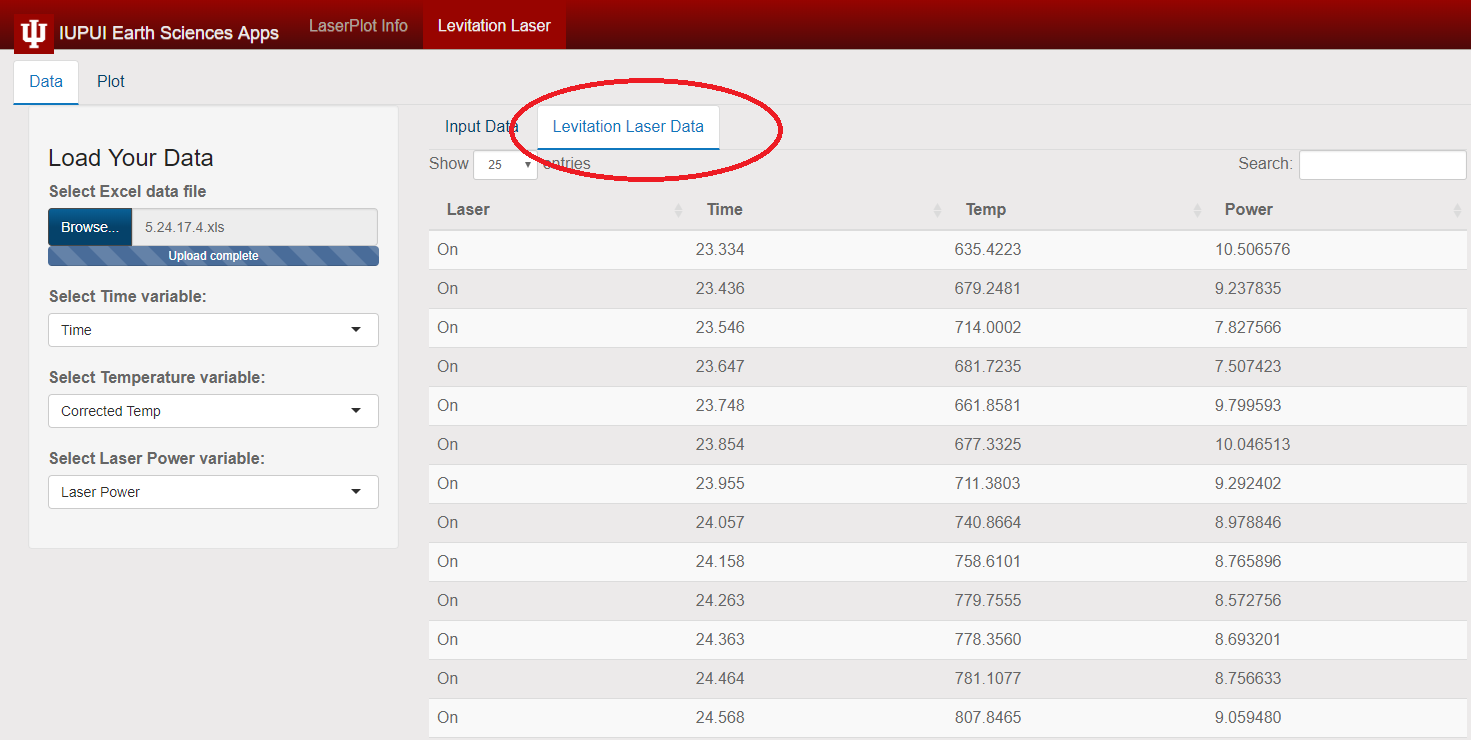The Levitation Laser Data tab shows the variables which will be represented by the x and y axes. This subset of the data is broken into “On” and “Off” values under the heading of Laser. This represents the time where the laser is turned on and the sample is heating and where the laser is turned off and the sample is supercooling.

### Plot Controls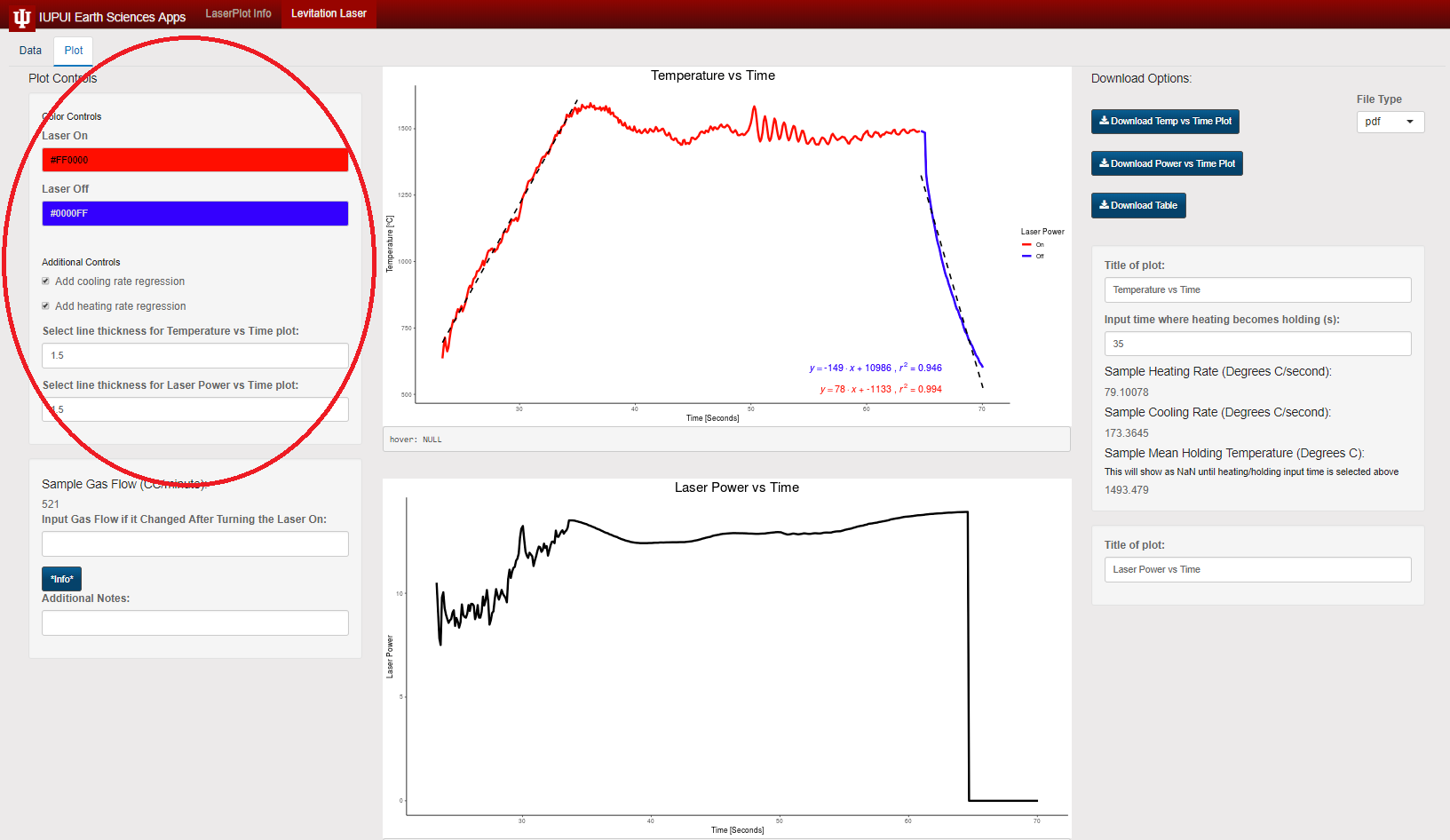By clicking on the colored boxes here, the color of the laser “On” and “Off” segments of the plot can be controlled. The checkbox will turn on/off the linear regression and accompanying formula on the plot. The next 2 input boxes allow for the adjustment of the line thicknesses for the 2 plots.

### Plot Titles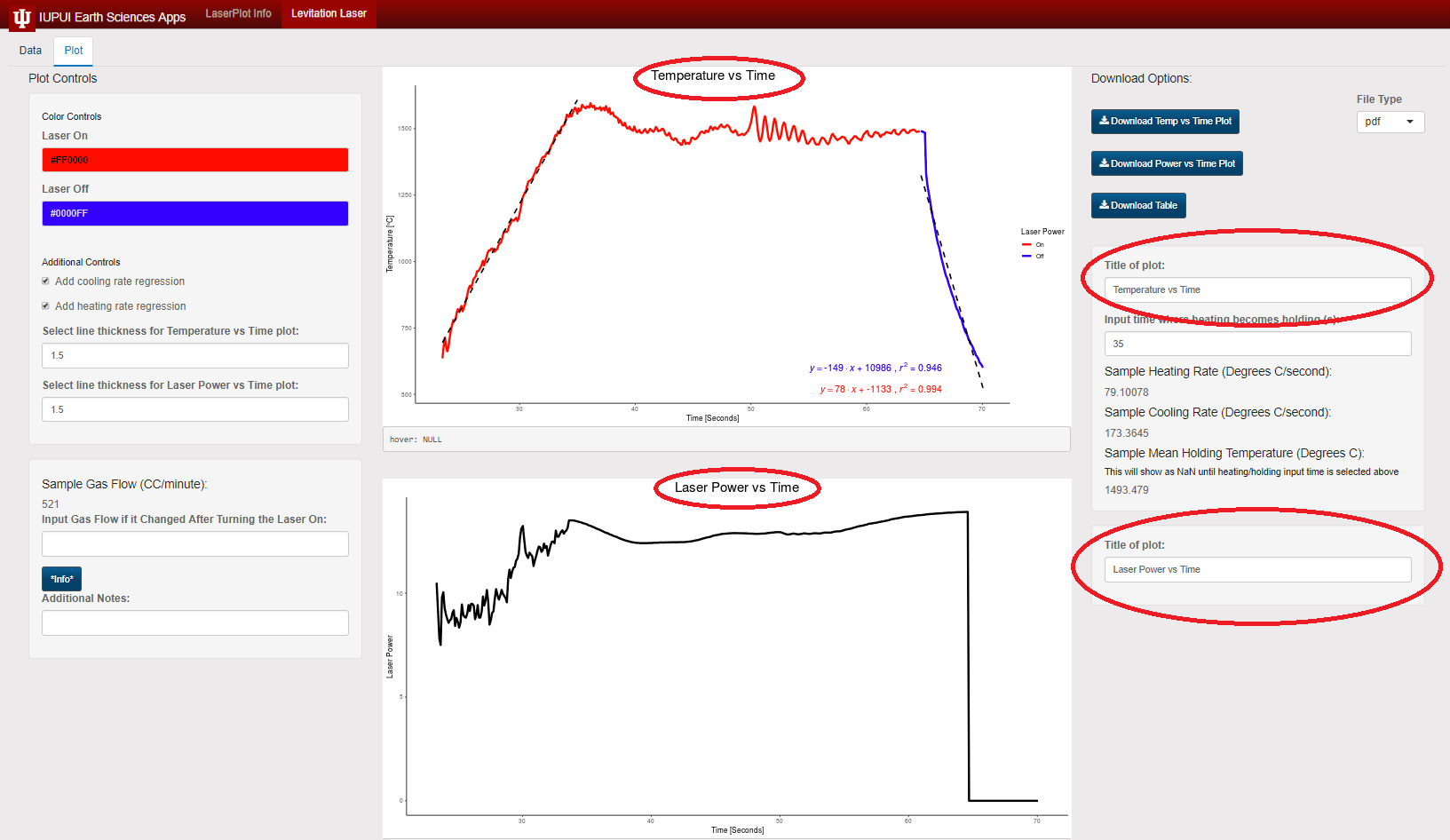The titles of the plot are automatically generated as Temperature vs Time and Laser Power vs Time. However, this can be adjusted to whatever is desired. It will adjust the title in real time.

### Plot Cooling and Heating Rates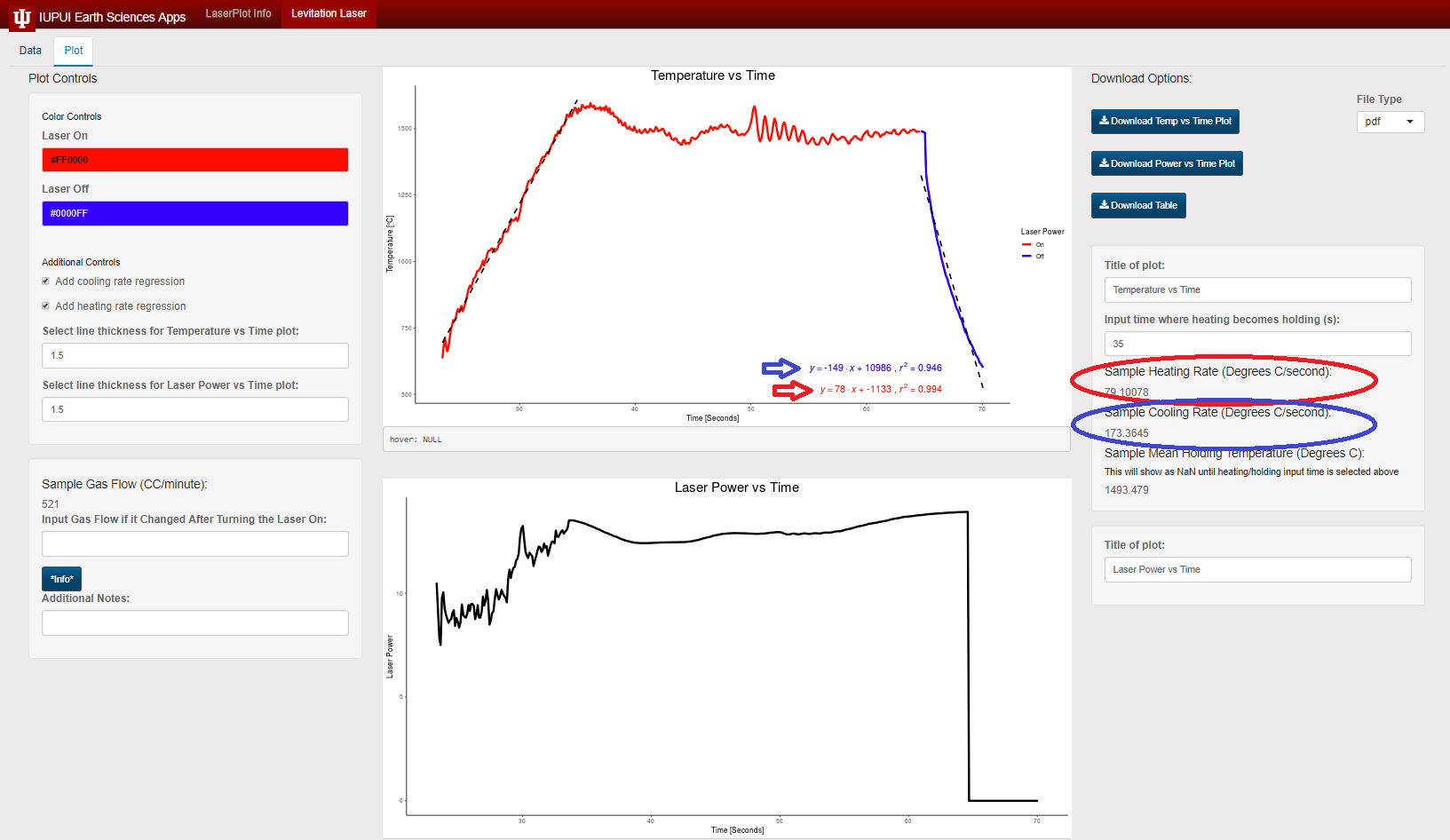These are the two separately calculated rates for sample cooling and heating. The slope of the linear regression formula calculates the rate of each by applying a best fit line, with the R-squared value included with the formula. The rates on the right are calculated by taking the derivative of temperature vs time at each increment, from the time that the laser is turned off until the temperature drops below 600 degrees Celsius. These derivatives are then averaged over that time period. The color of each equation matches the selected color of the “Laser On” and “Laser Off” controls.

### Controlling Duration for Heating Rate Calculations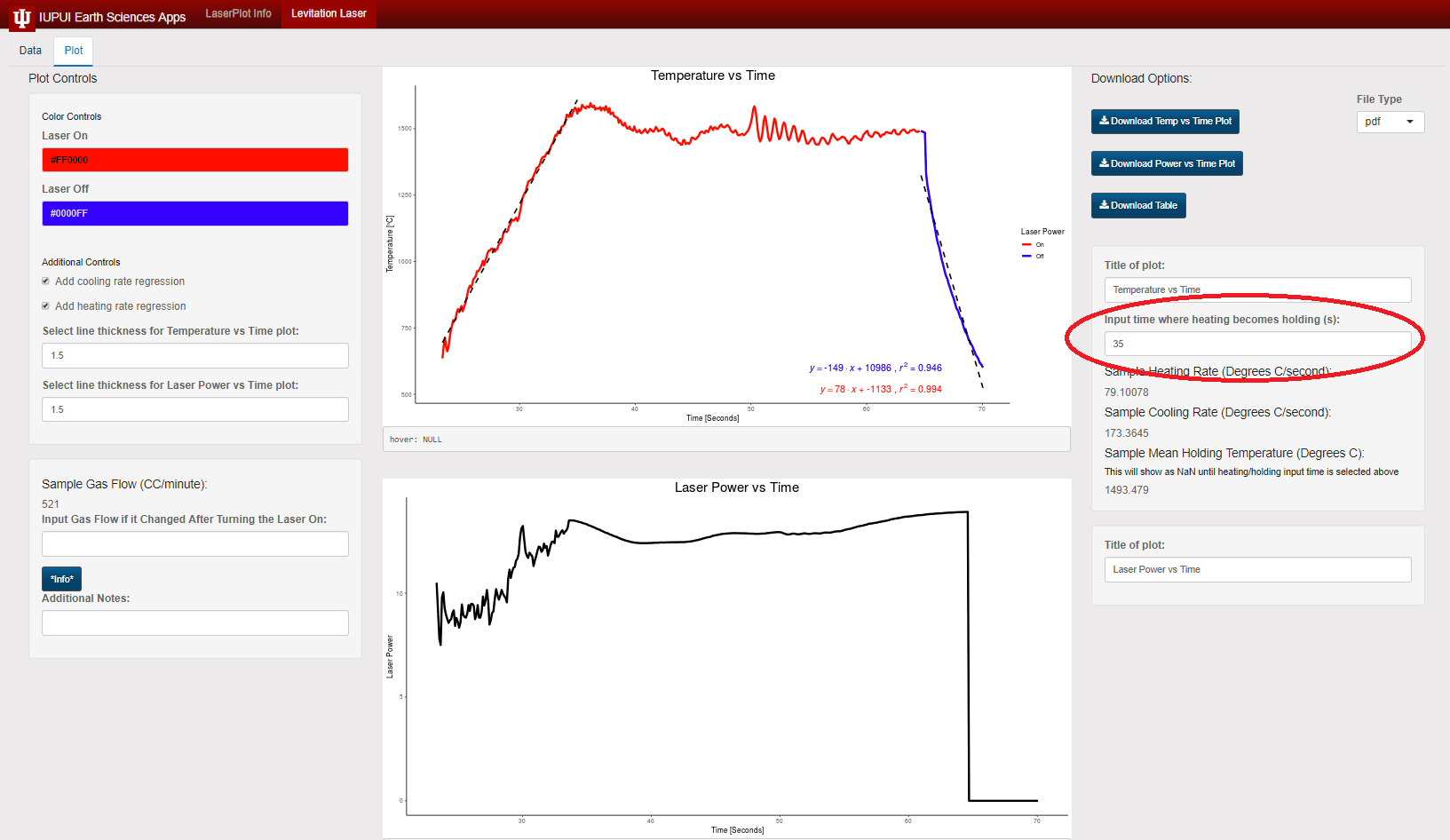This text box allows the user to input the time at which the sample is no longer heating. This could be the point at which the sample begins holding at/near a given temperature. Or irregular temperature fluctuations could be misrepresenting the heating rate. The plots have a function which shows the x and y coordiantes of the cursor (noted by “hover:” beneath the plot window). Find the desired location with the cursor, then input that time in this text box. The heat rate and linear regressions will update in real time.